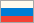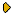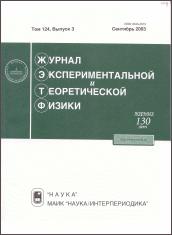Journal of Experimental and Theoretical Physics
 HOME | SEARCH | AUTHORS | HELPJournal IssuesGolden PagesAbout This journalAims and ScopeEditorial BoardManuscript SubmissionGuidelines for AuthorsManuscript StatusContactsDear users! "JETP" web site has moved to new address: http://jetp.ras.ru. Please update your bookmarks! Latest issue ZhETF, Vol. 164, No. 6, December 2023 ZhETF - Current volume 164 No 6, December 2023No 5, November 2023No 4, October 2023No 3, September 2023No 2, August 2023No 1, July 2023 Russian version archive (ZhETF) Vol. 164, 2023Vol. 163, 2023Vol. 162, 2022Vol. 161, 2022Vol. 160, 2021Vol. 159, 2021Vol. 158, 2020Vol. 157, 2020Vol. 156, 2019Vol. 155, 2019Vol. 154, 2018Vol. 153, 2018Vol. 152, 2017Vol. 151, 2017Vol. 150, 2016Vol. 149, 2016Vol. 148, 2015Vol. 147, 2015Vol. 146, 2014Vol. 145, 2014Vol. 144, 2013Vol. 143, 2013Vol. 142, 2012Vol. 141, 2012Vol. 140, 2011Vol. 139, 2011Vol. 138, 2010Vol. 137, 2010Vol. 136, 2009Vol. 135, 2009Vol. 134, 2008Vol. 133, 2008Vol. 132, 2007Vol. 131, 2007Vol. 130, 2006Vol. 129, 2006Vol. 128, 2005Vol. 127, 2005Vol. 126, 2004Vol. 125, 2004Vol. 124, 2003Vol. 123, 2003Vol. 122, 2002Vol. 121, 2002Vol. 120, 2001Vol. 119, 2001Vol. 118, 2000Vol. 117, 2000Vol. 116, 1999Vol. 115, 1999Vol. 114, 1998Vol. 113, 1998Vol. 112, 1997Vol. 111, 1997Vol. 110, 1996Vol. 109, 1996Vol. 108, 1995Vol. 107, 1995Vol. 106, 1994Vol. 105, 1994Vol. 104, 1993Vol. 103, 1993Vol. 102, 1992Vol. 101, 1992Vol. 100, 1991Vol. 99, 1991Vol. 98, 1990Vol. 97, 1990Vol. 96, 1989Vol. 95, 1989Vol. 94, 1988Vol. 93, 1987Vol. 92, 1987Vol. 91, 1986Vol. 90, 1986Vol. 89, 1985Vol. 88, 1985Vol. 87, 1984Vol. 86, 1984Vol. 85, 1983Vol. 84, 1983Vol. 83, 1982Vol. 82, 1982Vol. 81, 1981Vol. 80, 1981Vol. 79, 1980Vol. 78, 1980Vol. 77, 1979Vol. 76, 1979Vol. 75, 1978Vol. 74, 1978Vol. 73, 1977Vol. 72, 1977Vol. 71, 1976Vol. 70, 1976Vol. 69, 1975Vol. 68, 1975Vol. 67, 1975Vol. 66, 1974Vol. 65, 1974Vol. 64, 1973Vol. 63, 1973Vol. 62, 1972Vol. 61, 1972Vol. 60, 1971Vol. 59, 1971Vol. 58, 1970Vol. 57, 1970Vol. 56, 1969Vol. 55, 1969Vol. 54, 1968Vol. 53, 1968Vol. 52, 1967Vol. 51, 1967Vol. 50, 1966Vol. 49, 1966Vol. 48, 1965Vol. 47, 1965Vol. 46, 1964Vol. 45, 1964Vol. 44, 1963Vol. 43, 1963Vol. 42, 1962Vol. 41, 1962Vol. 40, 1961Vol. 39, 1961Vol. 38, 1960Vol. 37, 1960Vol. 36, 1959Vol. 35, 1959Vol. 34, 1958Vol. 33, 1958Vol. 32, 1957Vol. 31, 1957Vol. 30, 1956Vol. 29, 1956Vol. 28, 1955 English version archive (JETP, 1967-1996) Vol. 83, 1996Vol. 82, 1996Vol. 81, 1995Vol. 80, 1995Vol. 79, 1994Vol. 78, 1994Vol. 77, 1993Vol. 76, 1993Vol. 75, 1992Vol. 74, 1992Vol. 73, 1991Vol. 72, 1991Vol. 71, 1990Vol. 70, 1990Vol. 69, 1989Vol. 68, 1989Vol. 67, 1988Vol. 66, 1987Vol. 65, 1987Vol. 64, 1986Vol. 63, 1986Vol. 62, 1985Vol. 61, 1985Vol. 60, 1984Vol. 59, 1984Vol. 58, 1983Vol. 57, 1983Vol. 56, 1982Vol. 55, 1982Vol. 54, 1981Vol. 53, 1981Vol. 52, 1980Vol. 51, 1980Vol. 50, 1979Vol. 49, 1979Vol. 48, 1978Vol. 47, 1978Vol. 46, 1977Vol. 45, 1977Vol. 44, 1976Vol. 43, 1976Vol. 42, 1975Vol. 41, 1975Vol. 40, 1975Vol. 39, 1974Vol. 38, 1974Vol. 37, 1973Vol. 36, 1973Vol. 35, 1972Vol. 34, 1972Vol. 33, 1971Vol. 32, 1971Vol. 31, 1970Vol. 30, 1970Vol. 29, 1969Vol. 28, 1969Vol. 27, 1968Vol. 26, 1968Vol. 25, 1967Vol. 24, 1967Vol. 23, 1966Vol. 22, 1966Vol. 21, 1965Vol. 20, 1965Vol. 19, 1964Vol. 18, 1964Vol. 17, 1963Vol. 16, 1963Vol. 15, 1962Vol. 14, 1962Vol. 13, 1961Vol. 12, 1961Vol. 11, 1960Vol. 10, 1960Vol. 9, 1959Vol. 8, 1959Vol. 7, 1958Vol. 6, 1958Vol. 5, 1957Vol. 4, 1957Vol. 3, 1956Vol. 2, 1956Vol. 1, 1955 Accepted manuscripts Dear authors! In accordance with MAIK regulations, from September 1, 2016 manuscripts submitted in English will be translated in Russian for the Russian version of our journal.Print ISSN: 0044-4510
Report problems